Analysis WebNotes
arrow_back arrow_forward

# Class Contents

## Images and Preimages of Sets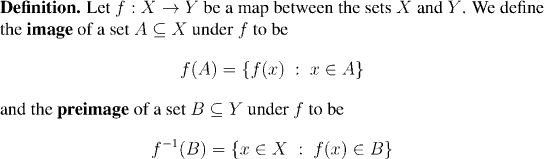Remark:

• The image of a set is the set of things that the elements of the set are mapped to.
• The preimage of a set is the set of things that are mapped into that set.
• Remember that both the image and the preimage of a set are themselves sets, not numbers.
• The following two criteria are very useful in constructing proofs involving images and preimages. It's always worth reminding yourself that for an element to belong to the image or preimage of a set means no more and no less than the following: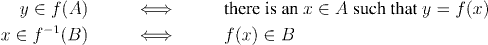Example:

Consider the function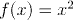which is a map of the real line to itself. Then: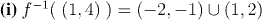Question: Prove it.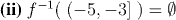Question: Prove it.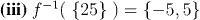Question: Prove it.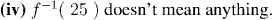Question: Explain this.

Now we come to the characterization of continuity in terms of open sets.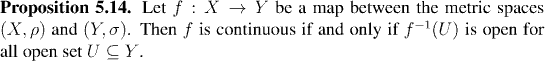Remark:

Note that the preimage of an open set under a continuous function is open, but that the image of an open set need not be. The next example illustrates this.

Example:

Again, letThen: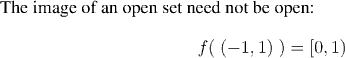Question: Prove it.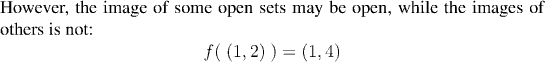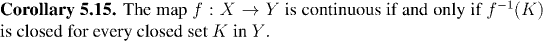The proof of the corollary is based on Proposition 5.12, coupled with the following fact about preimages:

Factoid:Remark:

By just the same token as before, the images of closed sets need not be closed. The images of some closed sets might be closed, but in general, continuous maps do not map closed sets to closed sets. (For example f(x)=1/(1+x^2) is continuous on the whole real line, but maps the set of natural numbers to a set which has 1 as a limit point, but does not contain it.)

There is however one important case in which closed sets are mapped to closed sets. All closed and bounded subsets of the real line share a property called compactness. The next chapter will be devoted to the study of compactness, and one of the things we'll see is that continuous functions always map compact sets to compact sets, and that compact sets are always closed (and bounded). Thus continuous functions will always map closed bounded sets in the real line to closed sets.